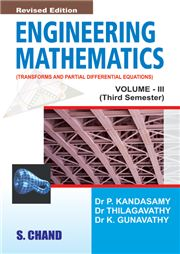# Engineering Mathematics Vol -III ( Tamil Nadu), 4/e P Kandasamy-S.CHAND

295.00 265.00

Chapter-I: Fourier Series Chapter-Ii:Partial Differential Equations Chapter-Iii:Applications Of Partial Differential Equations Boundary Value Problems Vibration Of Strings Heat Flow-One Dimension Heat Flow-Two Dimension (Cartesian) Heat Flow-Polar Equation